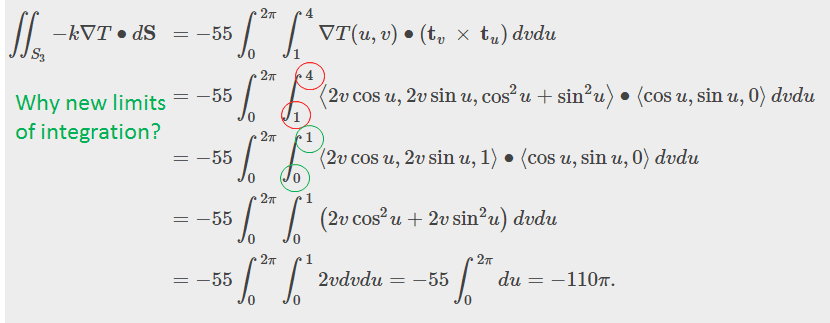# Surface integral: Calculate the heat flow from a cylinder

krihamm
Homework Statement:
Calculate the heat flow from a cylinder
Relevant Equations:
See below
Hi,

I am trying to calculate the heat flow across the boundary of a solid cylinder. The cylinder is described by x^2 + y^2 ≤ 1, 1 ≤ z ≤ 4. The temperature at point (x,y,z) in a region containing the cylinder is T(x,y,z) = (x^2 + y^2)z. The thermal conductivity of the cylinder is 55. The gradient of the temperature is nabla(T) = 2xzi + 2yzj + (x^2 + y^2)k.

I have calculated the heat flow across the bottom and top surfaces of the cylinder using the dot product of the gradient of T and the normal vector (e.g. the cross product of the tangent vectors in the u- and v-directions of the parameterized surface). They are 55pi/2 and -55pi/2, respectively. However, I am having trouble determining the heat flow across the cylindrical side.

According to the solutions manual (see figure below), the answer is -110pi. This is plausible based on the flow across the bottom and top surfaces of the cylinder. However, I do not understand the change of limits of integration in the solutions. I want to keep the limits as they are, but that results in a very high flow across the cylindrical side. Changing the limits of integration as the solution manual suggests yields a plausible answer, but I do not understand what justifies the change..

Any help is much appreciated!Best regards,
krihamm

Last edited by a moderator:

$$\int_1^4 2vdv = \int_0^1 2vdv$$